Three blocks A, B and C weighing 1, 8 and 27 kg respectively are connected as shown in the figure with an inextensible string and are moving on a smooth surface. T3 is equal to 36 N. Then T2 is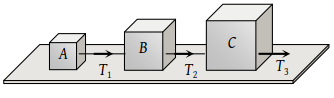(1) 18 N

(2) 9 N

(3) 3.375 N

(4) 1.25 N

Concept Questions :-

Newton laws
High Yielding Test Series + Question Bank - NEET 2020

Difficulty Level:

Two bodies of mass 3 kg and 4 kg are suspended at the ends of massless string passing over a frictionless pulley. The acceleration of the system is (g = 9.8 m/s2

(1) 4.9 m/s2

(2) 2.45 m/s2

(3) 1.4 m/s2

(4) 9.5 m/s2

Concept Questions :-

Application of laws
High Yielding Test Series + Question Bank - NEET 2020

Difficulty Level:

Three solids of masses m1, m2 and m3 are connected with weightless string in succession and are placed on a frictionless table. If the mass m3 is dragged with a force T, the tension in the string between m2 and m3 is

(1) $\frac{{m}_{2}}{{m}_{1}+{m}_{2}+{m}_{3}}T$

(2) $\frac{{m}_{3}}{{m}_{1}+{m}_{2}+{m}_{3}}T$

(3) $\frac{{m}_{1}+{m}_{2}}{{m}_{1}+{m}_{2}+{m}_{3}}T$

(4) $\frac{{m}_{2}+{m}_{3}}{{m}_{1}+{m}_{2}+{m}_{3}}T$

Concept Questions :-

Application of laws
High Yielding Test Series + Question Bank - NEET 2020

Difficulty Level:

Three blocks of masses m1, m2 and m3 are connected by massless strings as shown on a frictionless table. They are pulled with a force T3 = 40 N. If m1 = 10 kg, m2 = 6 kg and m3 4 kg, the tension T2 will be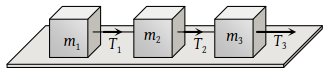(1) 20 N

(2) 40 N

(3) 10 N

(4) 32 N

Concept Questions :-

Types of forces
High Yielding Test Series + Question Bank - NEET 2020

Difficulty Level:

A light string passes over a frictionless pulley. To one of its ends a mass of 6 kg is attached. To its other end a mass of 10 kg is attached. The tension in the thread will be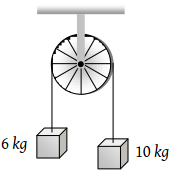(1) 24.5 N

(2) 2.45 N

(3) 79 N

(4) 73.5 N

Concept Questions :-

Application of laws
High Yielding Test Series + Question Bank - NEET 2020

Difficulty Level:

Two masses of 5kg and 10kg are connected to a pulley as shown. What will be the acceleration of the system (g = acceleration due to gravity)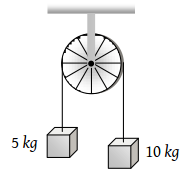(1) g

(2) $\frac{g}{2}$

(3) $\frac{g}{3}$

(4) $\frac{g}{4}$

Concept Questions :-

Application of laws
High Yielding Test Series + Question Bank - NEET 2020

Difficulty Level:

A block A of mass 7 kg is placed on a frictionless table. A thread tied to it passes over a frictionless pulley and carries a body B of mass 3 kg at the other end. The acceleration of the system is (given g = 10 ms–2)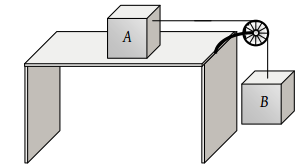(1) 100 ms–2

(2) 3 ms–2

(3) 10 ms–2

(4) 30 ms–2

Concept Questions :-

String constraint
High Yielding Test Series + Question Bank - NEET 2020

Difficulty Level:

Three blocks of masses 2 kg, 3 kg and 5 kg are connected to each other with light string and are then placed on a frictionless surface as shown in the figure. The system is pulled by a force F = 10 N, then tension T1 =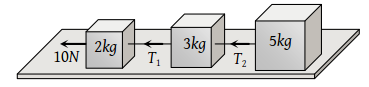(1) 1N

(2) 5 N

(3) 8 N

(4) 10 N

High Yielding Test Series + Question Bank - NEET 2020

Difficulty Level:

Two masses m1 and m2 are attached to a string which passes over a frictionless smooth pulley. When m1 = 10 kg, m2 = 6 kg, the acceleration of masses is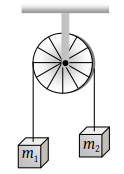(1) 20 m/s2

(2) 5 m/s2

(3) 2.5 m/s2

(4) 10 m/s2

Concept Questions :-

Application of laws
High Yielding Test Series + Question Bank - NEET 2020

Difficulty Level:

A body of weight 2kg is suspended as shown in the figure. The tension T1 in the horizontal string (in kg wt) is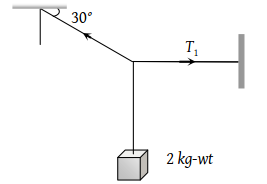(1) $2/\sqrt{3}$

(2) $\sqrt{3}/2$

(3) $2\sqrt{3}$

(4) 2

Concept Questions :-

Types of forces